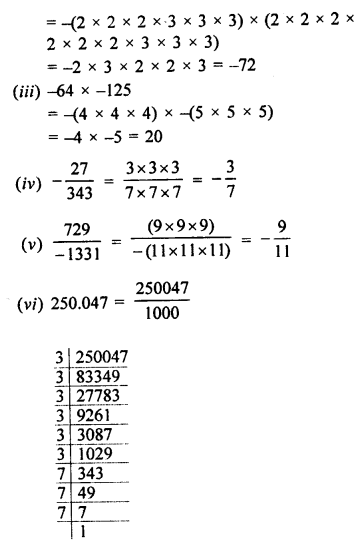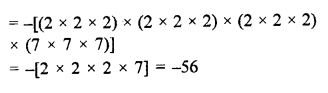## Selina Concise Mathematics Class 8 ICSE Solutions Chapter 4 Cubes and Cube-Roots (Including use of tables for natural numbers)

Selina Publishers Concise Mathematics Class 8 ICSE Solutions Chapter 4 Cubes and Cube-Roots (Including use of tables for natural numbers)

ICSESolutions.com provides step by step solutions for Selina Concise ICSE Solutions for Class 8 Mathematics Chapter 4 Cubes and Cube-Roots (Including use of tables for natural numbers). You can download the Selina Concise Mathematics ICSE Solutions for Class 8 with Free PDF download option. Selina Publishers Concise Mathematics for Class 8 ICSE Solutions all questions are solved and explained by expert mathematic teachers as per ICSE board guidelines.

### Cubes and Cube-Roots Exercise 4A – Selina Concise Mathematics Class 8 ICSE Solutions

Question 1.
Find the cube of :
(i) 7
(ii) 11
(iii) 16
(iv) 23
(v) 31
(vi) 42
(vii) 54
Solution:
(i) (7)3 = 7 x 7 x 7 = 343
(ii) (11)3 =11 x 11 x 11 = 1331
(iii) (16)3 = 16 x 16 x 16 = 4096
(iv) (23)3 = 23 x 23 x 23 = 12167
(v) (31)3 = 31 x 31 x 31 = 29791
(vi) (42)3 = 42 x 42 x 42 = 74088
(vii) (54)3 = 54 x 54 x 54 = 157464

Question 2.
Find which of the following are perfect cubes :
(i) 243
(ii) 588
(iii) 1331
(iv) 24000
(v) 1728
(vi) 1938
Solution: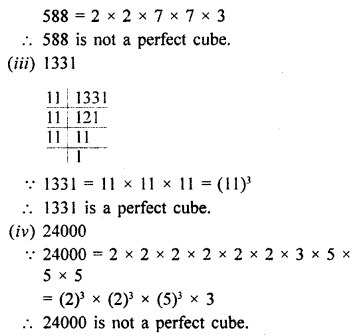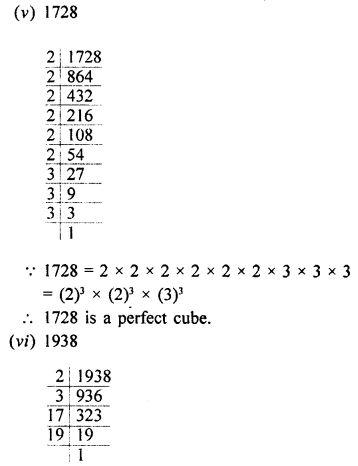1938 = 2 x 3 x 17 x 19
1938 is not a perfect cube.

Question 3.
Find the cubes of :
(i) 2.1
(ii) 0.4
(iii) 1.6
(iv) 2.5
(v) 0.12
(vi) 0.02
(vii) 0.8
Solution: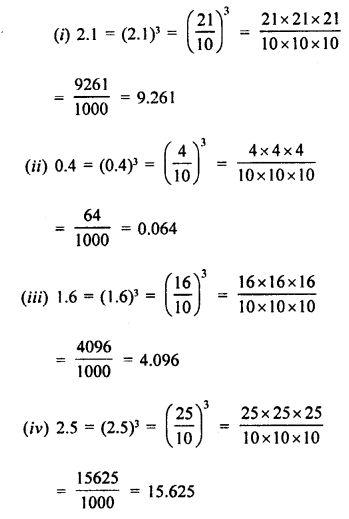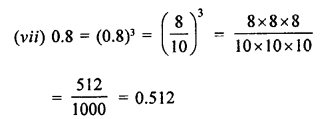Question 4.
Find the cubes of :
(i) $$\frac { 3 }{ 7 }$$
(ii) $$\frac { 8 }{ 9 }$$
(iii) $$\frac { 10 }{ 13 }$$
(iv) $$1\frac { 2 }{ 7 }$$
(v) $$2\frac { 1 }{ 2 }$$
Solution:Question 5.
Find the cubes of :
(i) -3
(ii) -7
(iii) -12
(iv) -18
(v) -25
(vi) -30
(vii) -50
Solution: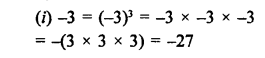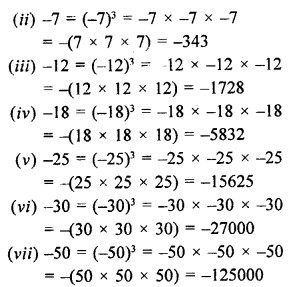Question 6.
Which of the following are cubes of:
(i) an even number
(ii) an odd number
216, 729, 3375, 8000, 125, 343, 4096 and 9261.
Solution: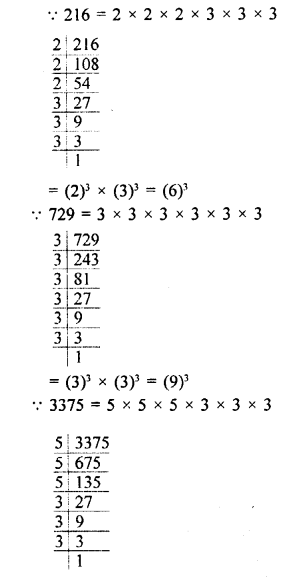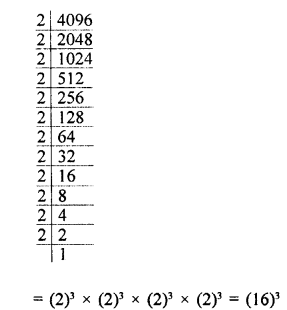(i) Cubes of an even number are 216, 8000, 4096.
(ii) Cubes of an odd number are 729, 3375, 125, 343, 9261.

Question 7.
Find the least number by which 1323 must be multiplied so that the product is a perfect cube.
Solution:
The prime factor of 1323 are =3 x 3 x 3 x 7 x 7
= (3 x 3 x 3) x 7 x 7
Clearly, 1323 must be multiplied by 7.

Question 8.
Find the smallest number by which 8768 must be divided so that the quotient is a perfect cube.
Solution: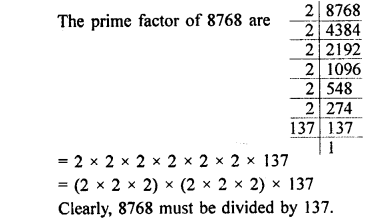Question 9.
Find the smallest number by which 27783 be multiplied to get a perfect square number.
Solution:Question 10.
With what least number must 8640 be divided so that the quotient is a perfect cube?
Solution:Question 11.
Which is the smallest number that must be multiplied to 77175 to make it a perfect cube?
Solution:### Cubes and Cube-Roots Exercise 4B – Selina Concise Mathematics Class 8 ICSE Solutions

Question 1.
Find the cube-roots of :
(i) 64
(ii) 343
(iii) 729
(iv) 1728
(v) 9261
(vi) 4096
(vii) 8000
(viii) 3375
Solution: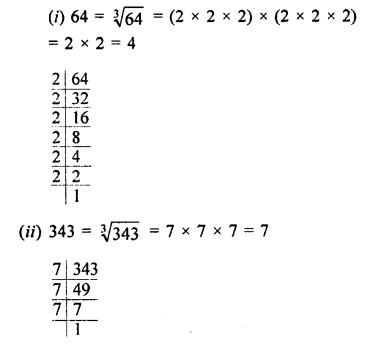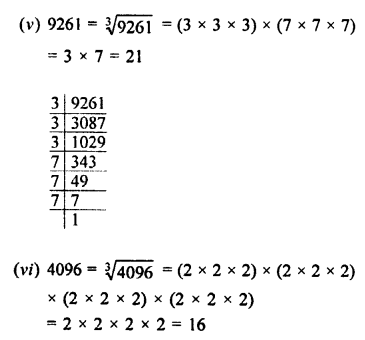Question 2.
Find the cube-roots of :
(i) $$\frac { 27 }{ 64 }$$
(ii) $$\frac { 125 }{ 216 }$$
(iii) $$\frac { 343 }{ 512 }$$
(iv) 64 x 729
(v) 64 x 27
(vi) 729 x 8000
(vii) 3375 x 512
Solution: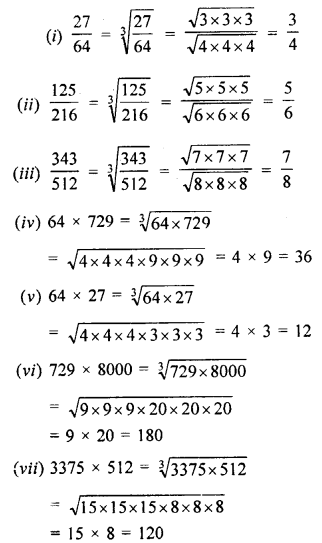Question 3.
Find the cube-roots of :
(i) -216
(ii) -512
(iii) -1331
(iv) $$\frac { -27 }{ 125 }$$
(v) $$\frac { -64 }{ 343 }$$
(vi) $$\frac { -512 }{ 343 }$$
(vii) -2197
(viii) -5832
(ix) -2744000
Solution: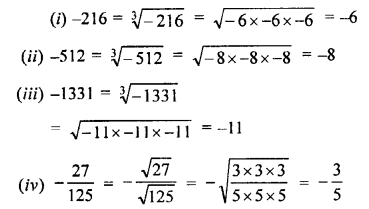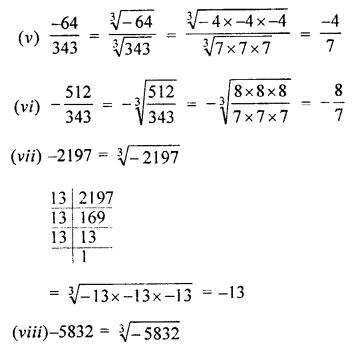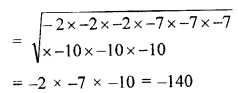Question 4.
Find the cube-roots of :
(i) 2.744
(ii) 9.261
(iii) 0.000027
(iv) -0.512
(v) -15.625
(vi) -125 x 1000
Solution: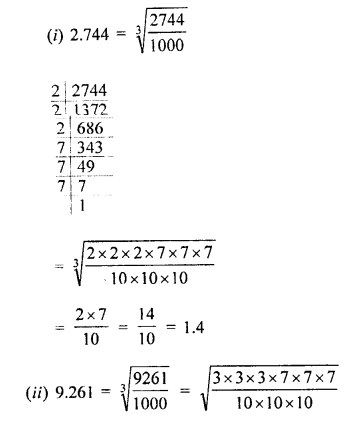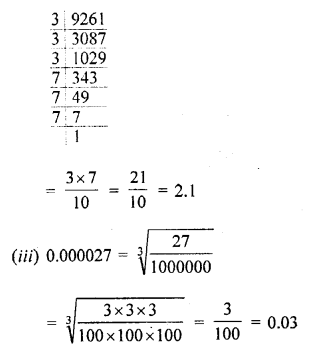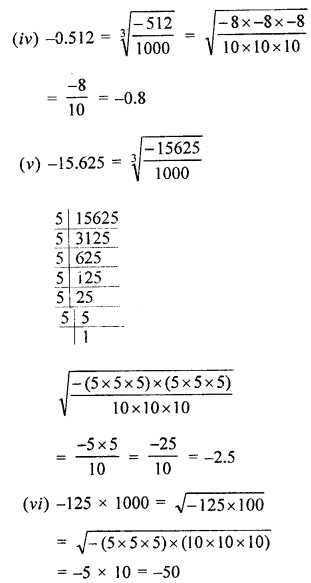Question 5.
Find the smallest number by which 26244 may be divided so that the quotient is a perfect cube.
Solution:
The prime factors of 26244 are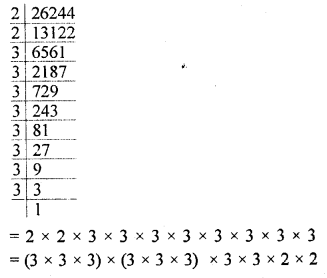Clearly, 26244 must be divided by
3 x 3 x 2 x 2 = 36

Question 6.
What is the least number by which 30375 should be multiplied to get a perfect cube?
Solution:
The prime factors of 30375 are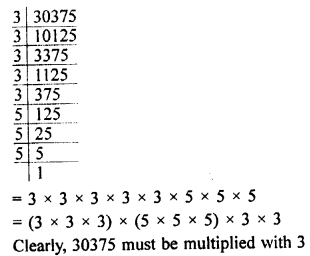Question 7.
Find the cube-roots of :
(i) 700 x 2 x 49 x 5
(ii) -216 x 1728
(iii) -64 x -125
(iv) $$\frac { -27 }{ 343 }$$
(v) $$\frac { 729 }{ -1331 }$$
(vi) 250.047
(vii) -175616
Solution: